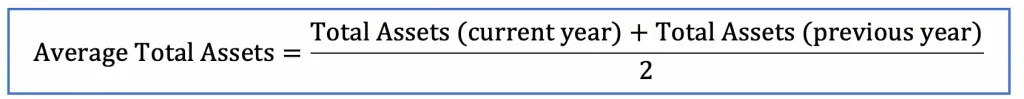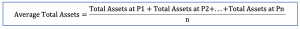# Average Total Assets

## Introduction

Average total assets are the average carrying value of assets that are recorded on the balance sheet at the different balance sheet dates. Usually, the carrying value of assets at the end of the previous year and those at the end of the current year are used in the calculation to find average total assets on the balance sheet.

The average total assets are usually used for calculation of asset turnover and return on asset ratio to measure the company’s ability in the utilization of the resources, its assets, to generate sales revenues and profit respectively.

## Average Total Assets Formula

Average total assets can be calculated by using total assets value at the end of the current year plus total assets value at the end of the previous year and then divide the result by two.Sometimes, total assets at the end of each month of the current year are used to find average total assets instead. In this case, all of the twelve total assets (of twelve months) are summed in total and divide by twelve to get average total assets.

However, this situation usually only happens in the management account where management accountant feels that the use of the average of twelve-month total assets gives more accurate results in evaluating the performance of the company.

In this case, the average total assets would be calculated as in formula below instead:where P = Period and n is the number of period, so

P1 = Period one

P2 = Period two

Pn= Period n

In this case, if we use average twelve months of total assets then n = 12 where P1 would be first month and P2 would be second month… and P12 would be 12th month.

## Average Total Assets Example

For example, we have the extracted balance sheet as below:

Extracted Balance Sheet 31 Dec 2019 31 Dec 2018
Current assets \$ \$
Cash and cash equivalents 11,296 14,862
Receivables 4,250 3,520
Inventories 10,396 8,580
25,942 26,962
Non-current assets
Equipment 60,782 51,640
Vehicles 87,500 87,500
Accumulated depreciation (19,387) (17,187)
128,895 121,953
Other assets 2,450 1,850
Total assets 157,287 150,765

What are the average total assets based on the balance sheet above?

## Calculate Average Total Assets

In the balance sheet above, we see that:

Total assets value at 31 Dec 2019 = \$157,287

Total assets value at 31 Dec 2018 = \$150,765

Then

Average Total Assets = (157,287 +150,765)/2 = \$154,026

So, the average total assets are \$154,026 which we can use to calculate various ratios, such as asset turnover and average return on assets.